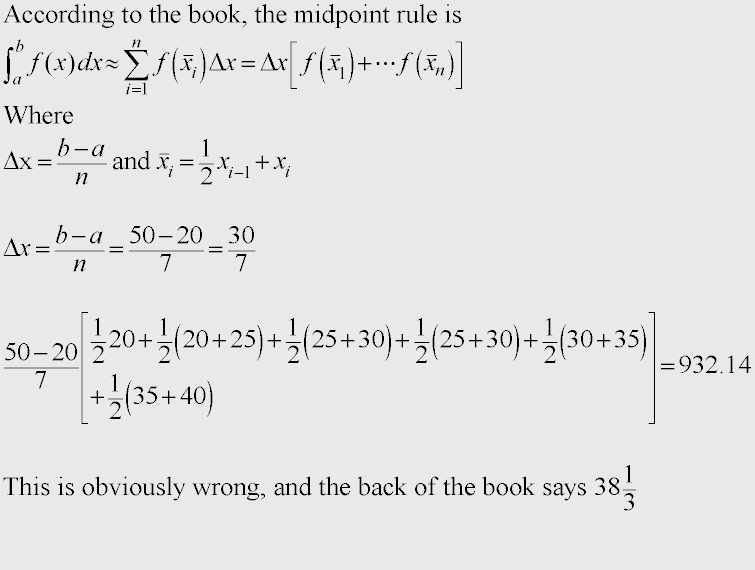# Estimating f on [20,50] with Midpoint Rule

• tony873004

#### tony873004

Gold Member
The table gives values of a continuous function. Use the Midpoint Rule to estimate the average value of f on [20, 50]

Code:
x      20  25   30   35   40   45   50
f(x)   42  38   31   29   35   48   60You were asked to find the "adverage value of the function over the interval".
Dont forget that to find this it is the intergral divided by the interval.

you also have your midpoint formula wrongly set up
delta x = (b - a)/n
so (50 - 20)/6

then you find the midpoints between the 7 f(x) values, note you used the x values in this step instead of the f(x) values

Midpoint sum = (50 -20)/60 * (.5(42 + 38) + .5(38 + 31) ...)

Now that you have the midpoint sum, or in other words the intergral of the function, divide that by the interval of 30. The anwser of 38.3333 is correct.

Thanks for the explanation.

(b-a)/n is what I have, but I used 7 and you used 6. I guess that's because they're being paired up, so its only 6 pairings?

But then you use (50-20)/60 instead of (50-20)/6. Where did the 60 come from?

When I punch this into the calculator, I get
(50-20)/60 * (.5*(42+38)+.5*(38+31)+.5*(31+29)+.5*(29+35)+.5*(35+48)+.5*(48+60))
which equals 116.

Edit ***
I forgot to divide by 30. If I use (50-20)/6 instead of 60, and divide by 30 I get 38.66667, instead of 38.33333. I'm guessing the 60 was a typo? I'm still off by 1/3

Last edited:
Last edited: